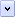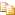Operator precedence is a set of rules in JScript. It controls the order in which operations are performed when an expression is evaluated. Operations with a higher precedence are performed before those with a lower one. For example, multiplication is performed before addition.

# JScript Operators

The following table lists the JScript operators, ordered from highest to lowest precedence. Operators with the same precedence are evaluated left to right.

Operator Description

. [] ()

Field access, array indexing, function calls, and expression grouping

++ -- - ~ ! delete new typeof void

Unary operators, return data type, object creation, undefined values

* / %

Multiplication, division, modulo division

+ - +

<< >> >>>

Bit shifting

< <= > >= instanceof

Less than, less than or equal, greater than, greater than or equal, instanceof

== != === !==

Equality, inequality, strict equality, and strict inequality

&

Bitwise AND

^

Bitwise XOR

|

Bitwise OR

&&

Logical AND

||

Logical OR

?:

Conditional

= OP=

Assignment, assignment with operation

,

Multiple evaluation

Parentheses are used to alter the order of evaluation determined by operator precedence. This means an expression within parentheses is fully evaluated before its value is used in the remainder of the expression.

For example:Copy Code
`z = 78 * (96 + 3 + 45)`

There are five operators in this expression: =, *, (), +, and another +. According to the rules of operator precedence, they are evaluated in the following order: (), +, +, *, =.

1. Evaluation of the expression within the parentheses occurs first. Within the parentheses, there are two addition operators. Since the addition operators both have the same precedence, they are evaluated from left to right. 96 and 3 are added together first, then 45 is added to this total, resulting in a value of 144.

2. Multiplication occurs next. 78 is multiplied by 144, resulting in a value of 11232.

3. Assignment occurs last. 11232 is assigned to z.

Home